Theory and Modern Applications

# Lie symmetry analysis of the time-variable coefficient B-BBM equation

## Abstract

We perform Lie group classification of a time-variable coefficient combined Burgers and Benjamin-Bona-Mahony equations (B-BBM equation). The direct analysis of the determining equations is employed to specify the forms of these time-dependent coefficients also known as arbitrary parameters. It is established that these model parameters have time-dependent functional forms of linear, power and exponential type.

## 1 Introduction

The Benjamin-Bona-Mahony equation, also known as the regularized-long-wave equation ,

${u}_{t}+{u}_{x}+u{u}_{x}-\delta {u}_{xxt}=0,$
(1)

boasts a wide range of applications in the unidirectional propagation of weakly long dispersive waves in inviscid fluids. Likewise, the Burgers equation 

${u}_{t}+u{u}_{x}+{u}_{xx}=0$
(2)

in its different forms has been studied extensively as a model of shock-waves in applications ranging from traffic flow to turbulence. The diverse approaches, both analytical and numerical, have been employed in the extensive studies of these equations in their various forms. Many of these investigations involve assuming the forms of the arbitrary parameters which appear in the mathematical models. However, the Lie group classification is a systematic approach through which the parameters assume their forms naturally. This is the essence of Lie group analysis of differential equations . The direct method of group classification [6, 7] is used to analyze the classifying relations, i.e., the equations which contain the arbitrary model parameters.

We study the one-dimensional modified B-BBM equation with power law nonlinearity and time-dependent coefficients given by 

${u}_{t}+f\left(t\right){u}^{q}{u}_{x}+g\left(t\right){u}_{xx}+h\left(t\right){u}_{xxt}=0,$
(3)

where $f\left(t\right)$, $g\left(t\right)$ and $h\left(t\right)$ are nonzero arbitrary elements and q is a positive constant. We deduce the following from Eq. (3): If $q=1$ and $h\left(t\right)=0$, then we obtain the variable coefficient Burgers equation

${u}_{t}+f\left(t\right)u{u}_{x}+g\left(t\right){u}_{xx}=0.$
(4)

A thorough investigation of the Lie group properties and some exact solutions of Eq. (4) can be found in .

On the other hand, the case $q=1$, $f\left(t\right)=1$, $h\left(t\right)=-\delta$ and $g\left(t\right)=0$ reduces Eq. (3) to the well-known equal width equation 

${u}_{t}+u{u}_{x}-\delta {u}_{xxt}=0.$
(5)

The outline of this work is as follows. The determining equations for the arbitrary elements (classifying relations) are generated in Section 2. In Section 3, the arbitrary elements are specified via the direct method of group classification. The results of group classification are utilized for symmetry reductions solutions in Section 4. Finally, we summarize our findings in Section 5.

## 2 Classifying equations and principal Lie algebra

The manual task of generating determining equations is tedious, but nowadays the Lie’s algorithm is implemented using a lot of various computer software packages for symbolic computation. We use the Mathematica software package YaLie  to generate and simplify the determining equations of the underlying Eq. (3) for maximal symmetry Lie algebra.

We look for the symmetry generator of Eq. (3) given by

$X={\xi }^{1}\left(t,x,u\right){\partial }_{t}+{\xi }^{2}\left(t,x,u\right){\partial }_{x}+\eta \left(t,x,u\right){\partial }_{u}.$
(6)

The coefficients of symmetry generator (6), namely ${\xi }^{1}$, ${\xi }^{2}$ and η, satisfy the determining equations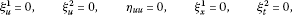(7)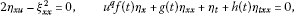(8)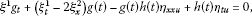(9)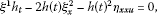(10)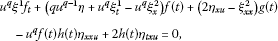(11)

where the subscripts denote partial differentiation with respect to the indicated variables.

We require that the arbitrary elements be nonzero, thus, the manipulation of Eqs. (7)-(11) yields the following forms of the coefficients of symmetry generator:

${\xi }^{1}={\xi }^{1}\left(t\right),\phantom{\rule{2em}{0ex}}{\xi }^{2}={c}_{1}x+{c}_{2},\phantom{\rule{2em}{0ex}}\eta =\left({c}_{3}+\frac{1}{2}{c}_{1}\right)u,$
(12)

where ${c}_{1}$, ${c}_{2}$ and ${c}_{3}$ are arbitrary constants. Upon back-substitution of (12) into the determining equations (7)-(11), we obtain the classifying relations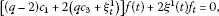(13)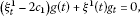(14)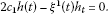(15)

Assume that the parameters $f\left(t\right)$, $g\left(t\right)$ and $h\left(t\right)$ are arbitrary smooth functions of t. Then from the last equations (13)-(15), we have

$\left(q-2\right){c}_{1}+2\left(q{c}_{3}+{\xi }_{t}^{1}\right)=0,\phantom{\rule{1em}{0ex}}{\xi }^{1}=0,{\xi }_{t}^{1}-2{c}_{1}=0,{c}_{1}=0.$
(16)

Upon solving (16), the coefficients of symmetry generator (12) become ${\xi }^{1}=0$, ${\xi }^{2}={c}_{2}$ and $\eta =0$. Therefore, we obtain a one-dimensional principal Lie algebra which is spanned by the operator ${X}_{1}={\partial }_{x}$.

## 3 Lie group classification

This section deals with specifying the forms of the arbitrary parameters through the direct analysis of the classifying relations with the aim of extending the principal symmetry Lie algebra.

We analyze the classifying relations (13)-(15) for the cases: $f\left(t\right)\ne 0$, $g\left(t\right)\ne 0$, $h\left(t\right)\ne 0$. In addition, we must have ${\xi }^{1}\left(t\right)\ne 0$ in order to extend the principal Lie algebra.

Case 1. $f\left(t\right)\ne 0$.

The classifying equation (13) can be written as

(17)

In solving (17), we consider the subcases: $m\ne 0$ and $m=0$.

Subcase 1.1. Suppose that $m\ne 0$, then from Eq. (17) we obtain

$f\left(t\right)={f}_{0}{e}^{mt},\phantom{\rule{2em}{0ex}}{\xi }^{1}\left(t\right)={c}_{4}{e}^{-mt}-\frac{\left(q-2\right){c}_{1}+2q{c}_{3}}{2m},$
(18)

where ${f}_{0}\ne 0$ and ${c}_{4}$ are arbitrary constants.

The other arbitrary functions get specified through the remaining classifying equations (14) and (15). If ${c}_{1}\ne 0$, then $g\left(t\right)$ and $h\left(t\right)$ assume the forms(19)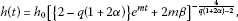(20)

where ${c}_{3}=\alpha {c}_{1}$, ${c}_{4}=\beta {c}_{1}$ and ${g}_{0}$, ${h}_{0}$ are nonzero arbitrary constants. The coefficients of symmetry generator (12) become

${\xi }^{1}=\beta {c}_{1}{e}^{-mt}-\frac{\left[q\left(1+2\alpha \right)-2\right]{c}_{1}}{2m},\phantom{\rule{2em}{0ex}}{\xi }^{2}=\alpha {c}_{1}x+{c}_{2},\phantom{\rule{2em}{0ex}}\eta =\frac{1}{2}\left(1+2\alpha \right)u{c}_{1}.$
(21)

It is established already that ${\partial }_{x}$ is the admitted generator, thus, we let ${c}_{2}=0$ without loss of generality. Therefore, the extension of the principal Lie algebra is given by the operator

${X}_{2}=\left[\left(2-q\left(1+2\alpha \right)\right)+2m\beta {e}^{-mt}\right]{\partial }_{t}+2mx{\partial }_{x}+m\left(1+2\alpha \right)u{\partial }_{u}.$
(22)

Now set $\alpha =\left(2-q\right)/2q$, then we have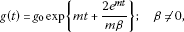(23)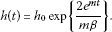(24)

The principal Lie algebra is extended by the operator

${X}_{2}=q\beta {e}^{-mt}{\partial }_{t}+qx{\partial }_{x}+u{\partial }_{u}.$
(25)

The condition: $\beta =0$ implies that $g\left(t\right)=0$ and $h\left(t\right)=0$ (this result is discarded).

If ${c}_{1}=0$, then from (18b) we obtain ${\xi }^{1}\left(t\right)={c}_{4}{e}^{-mt}-q{c}_{3}/m$. Thus, for ${c}_{4}\ne 0$, we have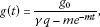(26)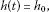(27)

where ${c}_{3}=\gamma {c}_{4}$. The operator which extends the principal Lie algebra is given by

${X}_{2}=\left(m{e}^{-mt}-\gamma q\right){\partial }_{t}+m\gamma u{\partial }_{u}.$
(28)

Now, for ${c}_{4}=0$, we obtain $g\left(t\right)={g}_{0}$ and $h\left(t\right)={h}_{0}$. Therefore, the extra operator is given by ${X}_{2}=q{\partial }_{t}-mu{\partial }_{u}$.

Subcase 1.2. Next assume that $m=0$, then solving Eq. (17) we obtain

$f\left(t\right)={\overline{f}}_{0},\phantom{\rule{2em}{0ex}}{\xi }^{1}\left(t\right)={c}_{5}+\frac{1}{2}t\left[\left(2-q\right){c}_{1}-2q{c}_{3}\right],$
(29)

where ${\overline{f}}_{0}\ne 0$ and ${c}_{5}$ are arbitrary constants. Suppose that ${c}_{1}\ne 0$, then $g\left(t\right)$ and $h\left(t\right)$ take the forms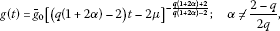(30)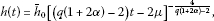(31)

where ${c}_{5}=\mu {c}_{1}$ and ${\overline{g}}_{0}$, ${\overline{h}}_{0}$ are arbitrary constants. The corresponding additional operator is given by

${X}_{2}=\left[\left(q\left(1+2\alpha \right)-2\right)t-2\mu \right]{\partial }_{t}-2x{\partial }_{x}-\left(1+2\alpha \right)u{\partial }_{u}.$
(32)

Now let $\alpha =\left(2-q\right)/2q$, then we obtain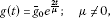(33)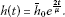(34)

Therefore, the principal symmetry Lie algebra is extended by the operator

${X}_{2}=q\mu {\partial }_{t}+qx{\partial }_{x}+u{\partial }_{u}.$
(35)

We obtain $g\left(t\right)=h\left(t\right)=0$ for $\mu =0$ (this result is excluded).

Now, if ${c}_{1}=0$, then from (17b) we get ${\xi }^{1}\left(t\right)={c}_{5}-q{c}_{3}t$. Thus, we obtain the following forms for $g\left(t\right)$ and $h\left(t\right)$: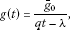(36)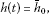(37)

where ${c}_{5}=\lambda {c}_{3}$ provided ${c}_{3}\ne 0$. The additional operator to the principal Lie algebra is given by

${X}_{2}=\left(\lambda -qt\right){\partial }_{t}+u{\partial }_{u}.$
(38)

The condition ${c}_{3}=0$ implies that $g\left(t\right)={\overline{g}}_{0}$ and $h\left(t\right)={\overline{h}}_{0}$ which corresponds to a constant coefficient case. The principal Lie algebra is extended by the operator ${X}_{2}={\partial }_{t}$.

Case 2. Next we consider the case $g\left(t\right)\ne 0$. Proceeding as in the previous case, here we base our analysis on the classifying equation (14). As a result, we obtain the following classification results.

Subcase 2.1. For some constant $n\ne 0$, we have $g\left(t\right)={\stackrel{˜}{g}}_{0}{e}^{nt}$ and thus,

2.1.1. ${c}_{1}\ne 0$.(39)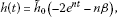(40)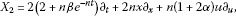(41)

where ${\stackrel{˜}{f}}_{0}$, ${\stackrel{˜}{g}}_{0}$ and ${\stackrel{˜}{h}}_{0}$ are nonzero arbitrary constants.

2.1.2. ${c}_{1}=0$ (${c}_{4}\ne 0$).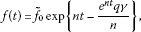(42)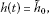(43)(44)

Subcase 2.2. $n=0$: $g\left(t\right)={\stackrel{˜}{g}}_{0}$.

2.2.1. ${c}_{1}\ne 0$.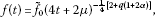(45)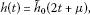(46)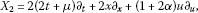(47)

where ${\stackrel{˜}{f}}_{0}$, ${\stackrel{˜}{g}}_{0}$ and ${\stackrel{˜}{h}}_{0}$ are nonzero arbitrary constants.

2.2.2. ${c}_{1}=0$ (${c}_{5}\ne 0$).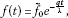(48)(49)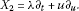(50)

Case 3. Lastly, we consider $h\left(t\right)\ne 0$. For a given constant k, the analysis of the classifying equation (15) leads to $h\left(t\right)={\stackrel{ˆ}{h}}_{0}{e}^{kt}$, where ${\stackrel{ˆ}{h}}_{0}\ne 0$. The corresponding forms of $f\left(t\right)$ and $g\left(t\right)$ together with the operator, which extends the principal Lie algebra, read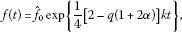(51)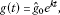(52)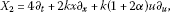(53)

where ${\stackrel{ˆ}{f}}_{0}\ne 0$ and ${\stackrel{ˆ}{g}}_{0}\ne 0$ are arbitrary constants. The last set of classification results is a particular case of 2.1.1 for some choice of arbitrary constants. It can also be shown by similar calculations that the remaining subcases are duplication of some subcases in Cases 1 and 2.

## 4 Symmetry reductions

Since the maximal symmetry Lie algebra is two-dimensional, the derivation of invariant solutions amounts to finding solutions invariant under the linear combination $c{X}_{1}+{X}_{2}$ where c is an arbitrary constant. For illustration, we consider Subcase 2.2. Firstly, we look at the Subcase 2.2.1. Thus, for $c\ne 0$, we have the linear combination

$c{X}_{1}+{X}_{2}=2\left(2t+\mu \right){\partial }_{t}+\left(2x+c\right){\partial }_{x}+\left(1+2\alpha \right)u{\partial }_{u}.$
(54)

The solution of the characteristic equation for (54) is given by the similarity solution

$u\left(t,x\right)={\left(4t+2\mu \right)}^{\frac{1}{4}\left(1+2\alpha \right)}F\left(z\right),$
(55)

where $F\left(z\right)$ is an arbitrary function of the similarity variable

$z=\frac{c+2x}{2\sqrt{2t+\mu }}.$

Upon substitution of the corresponding arbitrary parameters and the solution (55) into Eq. (3), we obtain the ordinary differential equation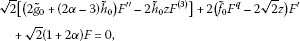(56)

where a ‘prime’ denotes derivative with respect to z.

If $c=0$, then the similarity variable takes the form $z=x/\sqrt{2t+\mu }$, but the similarity solution and the reduced equation remain the same.

Secondly, we consider the Subcase 2.2.2. Proceeding likewise for $c\ne 0$, the invariant solution assumes the form

$u\left(t,x\right)={e}^{t/\lambda }F\left(z\right),$
(57)

where $z=x-ct/\lambda$ is the similarity variable and $F\left(z\right)$ is an arbitrary function. The reduced equation is of the form

$F+\left(\lambda {\stackrel{˜}{f}}_{0}{F}^{q}-c\right){F}^{\prime }+\left({\stackrel{˜}{f}}_{0}+\lambda {\stackrel{˜}{g}}_{0}\right){F}^{″}-{\stackrel{˜}{h}}_{0}c{F}^{\left(3\right)}=0.$
(58)

Next, let $c=0$, then the similarity variable is given by $z=x$, and thus the similarity solution takes the form

$u\left(t,x\right)={e}^{t/\lambda }F\left(x\right),$
(59)

where F is an arbitrary function of its argument. The corresponding reduced equation is given by

$F+\lambda {\stackrel{˜}{f}}_{0}{F}^{q}{F}^{\prime }+\left({\stackrel{˜}{h}}_{0}+\lambda {\stackrel{˜}{g}}_{0}\right){F}^{″}=0.$
(60)

Remark It is noted that the reduced ordinary differential equations are still highly nonlinear to solve exactly.

## 5 Conclusion

The direct analysis of the classifying relations was employed to obtain the functional forms of the time-dependent arbitrary parameters for the B-BBM equation. These time-dependent functional forms are of linear, power and exponential type. The maximal symmetry Lie algebra of dimension two is obtained for each set of classification results. Consequently, the symmetries which span the symmetry Lie algebra are utilized to perform symmetry reductions.

## Dedication

This paper is dedicated to Prof. Ravi P. Agarwal on his 65th birth anniversary.

## References

1. Benjamin TB, Bona JL, Mahoney JJ: Model equations for long waves in nonlinear dispersive systems. Philos. Trans. R. Soc. Lond. 1972, 272: 47–78. 10.1098/rsta.1972.0032

2. Burgers JM: The Nonlinear Diffusion Equation. Reidel Publishing, Dordrecht; 1974.

3. Bluman GW, Kumei S: Symmetries and Differential Equations. Springer, New York; 1989.

4. Olver PJ: Applications of Lie Groups to Differential Equations. Springer, New York; 1986.

5. Ovsiannikov LV: Group Analysis of Differential Equations. Academic, New York; 1982.

6. Johnpillai AG, Khalique CM: Group analysis of KdV equation with time dependent coefficients. Appl. Math. Comput. 2010, 216: 3761–3771. 10.1016/j.amc.2010.05.043

7. Molati M, Ramollo MP: Symmetry classification of the Gardner equation with time-dependent coefficients arising in stratified fluids. Commun. Nonlinear Sci. Numer. Simul. 2012, 17: 1542–1548. 10.1016/j.cnsns.2011.09.002

8. Zhao Y, Zhang WG: Qualitative analysis and solutions of bounded travelling wave for B-BBM equation. Acta Math. Appl. Sin. 2010, 26: 415–426. 10.1007/s10255-010-0007-0

9. Sophocleous C: Transformation properties of a variable coefficient Burgers equation. Chaos Solitons Fractals 2004, 20: 1047–1057. 10.1016/j.chaos.2003.09.024

10. Morrison PJ, Meiss JD, Cary JR: Scattering of regularized-long-wave solitary waves. Physica D, Nonlinear Phenom. 1984, 11: 324–336. 10.1016/0167-2789(84)90014-9

11. Díaz, JM: Short guide to YaLie. Yet another Lie Mathematica package for Lie symmetries. http://library.wolfram.com/infocenter/MathSource/4231/

## Acknowledgements

CMK would like to thank the Organizing Committee of ‘International Conference on Applied Analysis and Algebra’, (ICAAA 2012) for their kind hospitality during the conference. MM thanks the North-West University, Mafikeng campus for the post-doctoral fellowship.

## Author information

Authors

### Corresponding author

Correspondence to Chaudry Masood Khalique.

### Competing interests

The authors declare that they have no competing interests.

### Authors’ contributions

MM and CMK worked together in the derivation of the mathematical results. Both authors read and approved the final manuscript.

## Rights and permissions

Reprints and Permissions

Molati, M., Khalique, C.M. Lie symmetry analysis of the time-variable coefficient B-BBM equation. Adv Differ Equ 2012, 212 (2012). https://doi.org/10.1186/1687-1847-2012-212